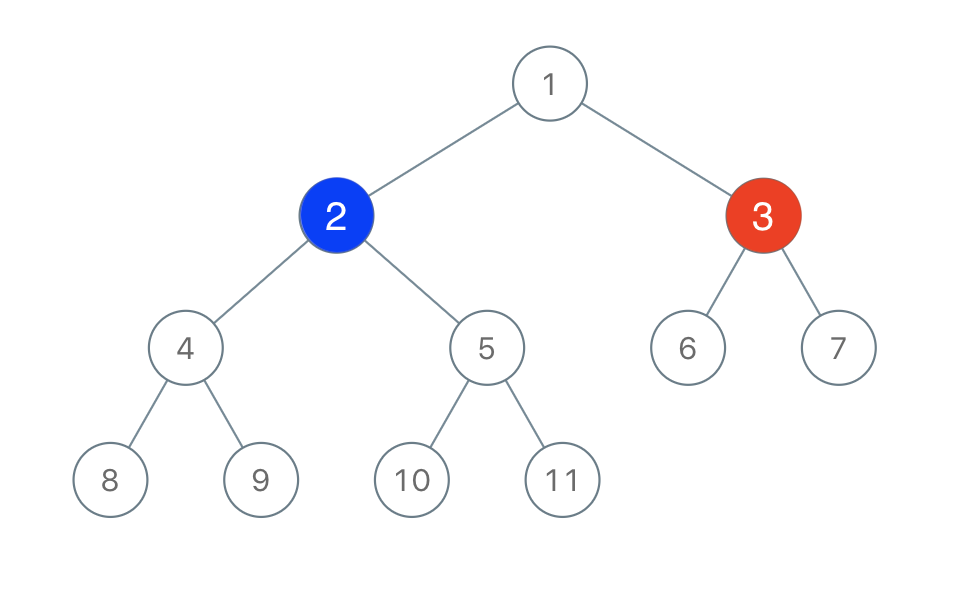# Binary Tree Coloring Game

Dec 27, 2019

• 除了第一个颜色可以随便找节点上色外，其他的都只能对自己临近的节点上色。
• 有颜色的节点不能再次上色
• 所以节点上完色后，节点多的人获胜bool btreeGameWinningMove(TreeNode* root, int n, int x) {
if (!root) return false;
TreeNode *node = search(root, x);

int leftNum = getNodeNum(node->left);
int rightNum = getNodeNum(node->right);
int upNum = n - 1 - leftNum - rightNum;
int maxNum = max(max(leftNum, rightNum), upNum);
// cout << leftNum << endl;
// cout << rightNum << endl;
// cout << upNum << endl;
// cout << maxNum << endl;
return maxNum > (n - maxNum);

}

int getNodeNum(TreeNode *root) {
if (!root) return 0;
return 1 + getNodeNum(root->left) + getNodeNum(root->right);
}

TreeNode *search(TreeNode *root, int x) {
if (!root || root->val == x) return root;
TreeNode *node = search(root->left, x);
if (node) return node;
return search(root->right, x);
}

LeetCodeLeetCode

Satisfiability of Equality Equations

Maximum Level Sum of a Binary Tree